# Help with Basic Circuit - KCL

• Engineering
• Fjolvar
So the 10 Ohm resistor is not necessary. In summary, the student is trying to solve a problem involving current and voltage, but is having difficulty. They are stuck and need help.

#### Fjolvar

I've only taken a basic circuits course and that was years ago. Can anyone help me get this one started?

1. Homework Statement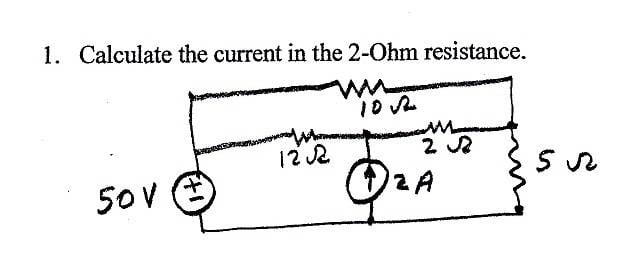KCL

## The Attempt at a Solution

[/B]
I'm not sure if I should use KCL right away or use Thevenin.

Since I need to find the current through the 2 ohm resistor, I can't combine the 10 ohm resistor with the 12 & 2 ohm resistor.
Also I'm not sure how the 2A current source would distribute through the circuit. Would the full 2A would flow through the 2 ohm resistor?

Any help would be much appreciated.

There's probably no "short cut" way that you are looking for. You'll have to work it out more formally:

You could let the current through the 2Ω be I1 and the current through some other resistor be I2. Gather equations based on KVL and KCL and solve for the two unknowns.

The method is to work out all branch currents and node voltages in terms of I1 and I2 and solve simultaneous equations to find the unknowns.

An alternative would be to use superposition: Suppress one source at a time and find the resulting current through the resistor, then sum the results. Suppressing either source presents opportunities for simplifying the circuit.

So I made an attempt using Thevenin and Superposition, but I got stuck early into it. I'm not quite sure how to find the Thevenin voltage with the 50V source in place and the current source removed. I'm not sure how I would calculate the voltage drop over a and b terminals since there is no resistor in parallel with it.

Looking over it now, I think my Rth is wrong too, since I realize that's not a shorted path.

Any ideas where I'm going wrong?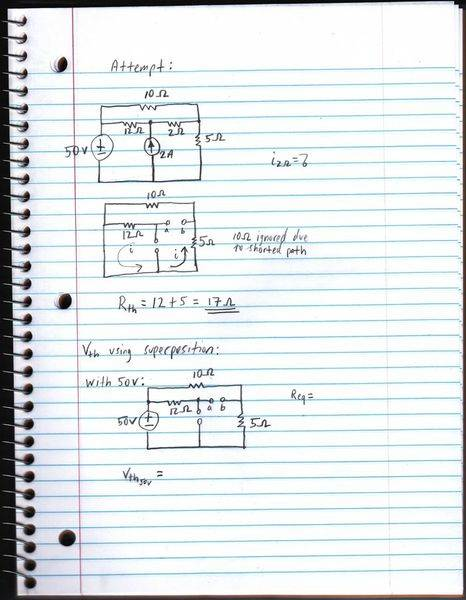Regarding your Thevenin Resistance, you can't ignore the 10 Ω resistor. Hint: slide the left end connection of the 10 Ω resistor along its node until it ends up at the bottom of the 5 Ω resistor. Now what do you see?

Regarding Vth, choose a reference node (say the bottom of the voltage supply) and determine the potentials at the a and b terminals separately. What then is Vab?

I made another attempt, but wanted to make sure I'm on the right track before I go any further.

Since there is no current flowing into the 12 ohm resistor branch, there is no voltage drop, right? Therefore I assumed Va = 0 and just calculated for the one loop containing the other two resistors and used voltage divider to find the voltage at terminal b.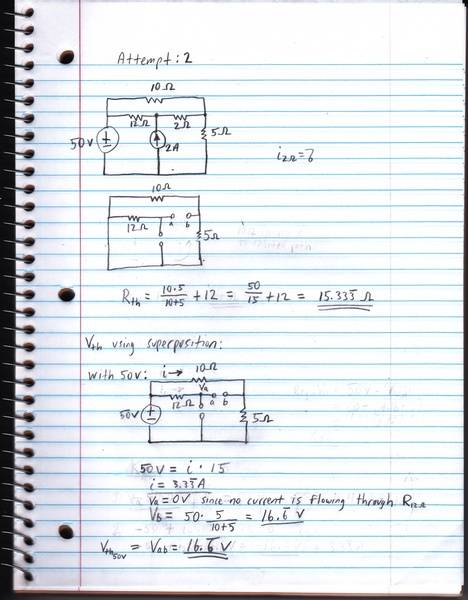Last edited:
Fjolvar said:
Since there is no current flowing into the 12 ohm resistor branch, there is no voltage drop, right? Therefore I assumed Va = 0 and just calculated for the one loop containing the other two resistors and used voltage divider to find the voltage at terminal b.
Nope. With no potential drop over the 12 Ohm resistor both ends of that resistor must be at the same potential. At what potential is the left end of that resistor?

gneill said:
Nope. With no potential drop over the 12 Ohm resistor both ends of that resistor must be at the same potential. At what potential is the left end of that resistor?

Looks like I got ahead of myself. So the voltage at terminal a is 50 V making Vab (50-16.6) = 33.4V, correct?

Last edited:
Fjolvar said:
Looks like I got ahead of myself. So the voltage at terminal a is 50 V making Vab (50-16.6) = 33.4V, correct?
Well, I get 33.3 V without rounding any intermediate steps. So yes, looks good.

Thanks gneill. I've moved onto the second part now with only the current source in place, but I'm still having trouble seeing what to do next. I've spent some time trying different approaches such as KVL and KCL, but it's not quite working out. I was hoping a current divider would be possible, but the resistors aren't in parallel. Could you give me a hint on this one? Btw this has b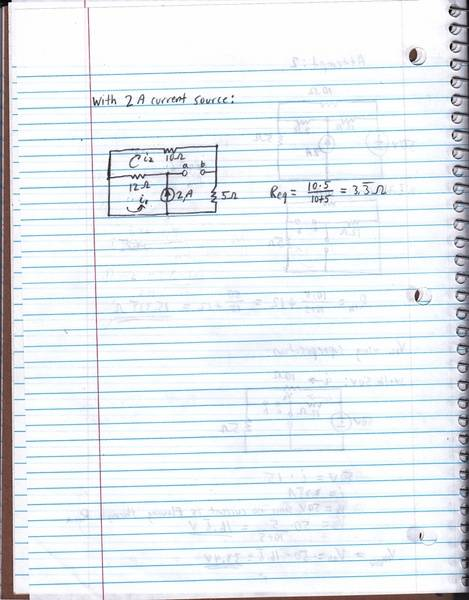Last edited:
Choose a reference node. The bottom of the current source looks appealing. Then move some of the resistors around a bit, keeping the same node connections (remember the trick with the 10 Ohm resistor that I pointed out previously?). You should reach an aha! moment.

So was the aha! moment supposed to be that i2 through the 10 ohm and 5 ohm resistor doesn't exist since the main current shorts back to the current source? Here is what I have for a final answer: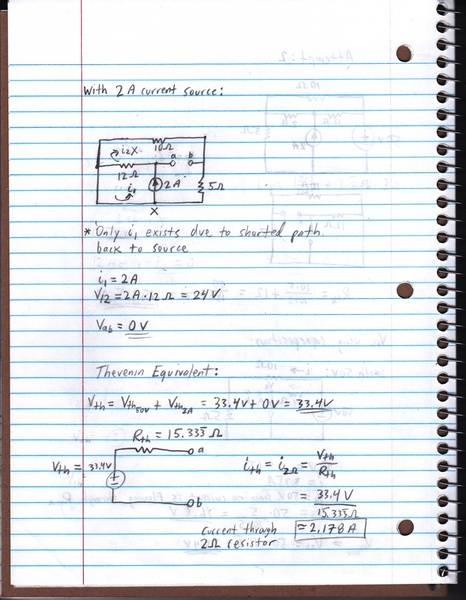Last edited:
Fjolvar said:
So was the aha! moment supposed to be that i2 through the 10 ohm and 5 ohm resistor doesn't exist since the main current shorts back to the current source?
More or less, yes. If you move the 10 Ohm resistor to parallel the 5 Ohm resistor then you can see that there's no closed loop for current to flow through either one. That makes the potential at terminal b the same as the potential of the reference node.
Here is what I have for a final answer: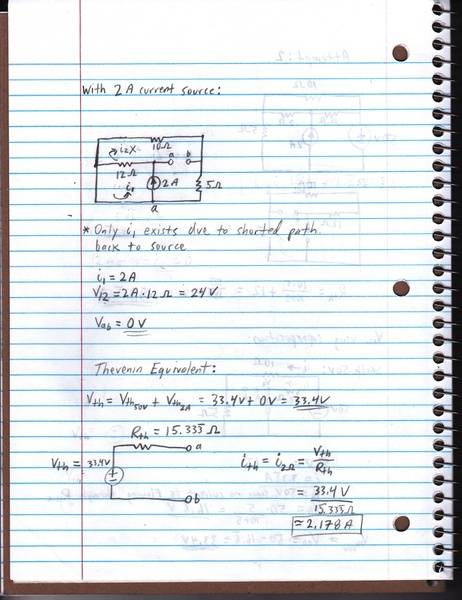You've written Vab = 0 V in your figure. That's not true, since there is a potential at b. Oh, hang on a moment, I see you've labelled the bottom node as a. Well, that's a bit confusing, having two different nodes with the same label.

Now, wasn't the objective to find the current through the 2 Ohm resistor? Where does it appear in your current calculation?

gneill said:
More or less, yes. If you move the 10 Ohm resistor to parallel the 5 Ohm resistor then you can see that there's no closed loop for current to flow through either one. That makes the potential at terminal b the same as the potential of the reference node.

You've written Vab = 0 V in your figure. That's not true, since there is a potential at b. Oh, hang on a moment, I see you've labelled the bottom node as a. Well, that's a bit confusing, having two different nodes with the same label.

Now, wasn't the objective to find the current through the 2 Ohm resistor? Where does it appear in your current calculation?

My mistake, I corrected the label of the node in the picture from 'a' to 'x'.

I assumed there is only a voltage dissipation across the 12 ohm resistor, but no voltage before at node a, since there's only a current source. Wouldn't the voltage at x also be zero? Since there's no voltage at node a and no current at node b (whose voltage equals the voltage at node x), it seems the voltage across terminals a and b is equal to zero when there's only a current source in place. I have a feeling I may have made an invalid assumption for this part.

Afterwards I just used Vth calculated when the 50V voltage source was in place with Rth to calculate the Thevenin current, which is the current through the 2 ohm resistor.

Fjolvar said:
My mistake, I corrected the label of the node in the picture from 'a' to 'x'.

I assumed there is only a voltage dissipation across the 12 ohm resistor, but no voltage before at node a, since there's only a current source. Wouldn't the voltage at x also be zero? Since there's no voltage at node a and no current at node b (whose voltage equals the voltage at node x), it seems the voltage across terminals a and b is equal to zero when there's only a current source in place. I have a feeling I may have made an invalid assumption for this part.
Yup. Taking the reference node as x, the potential at b is indeed the same as x, so 0 V with respect to the reference node. But terminal a has a potential with respect to the reference node due to the current through the 12 Ohm resistor.
Afterwards I just used Vth calculated when the 50V voltage source was in place with Rth to calculate the Thevenin current, which is the current through the 2 ohm resistor.
You've be better off determining the currents independently and summing them. You've created two distinct Thevenin models, one for each source.

For some reason I'm having trouble determining what the voltage at terminal a would be. Since we only know the voltage drop across the 12 ohm resistor and not the voltage before, I'm not quite sure what other information we can use.

What nodes does the 12 Ohm resistor connect to? Is one of them your reference node? The potential drop across that resistor must be the same as the potential difference between those nodes.